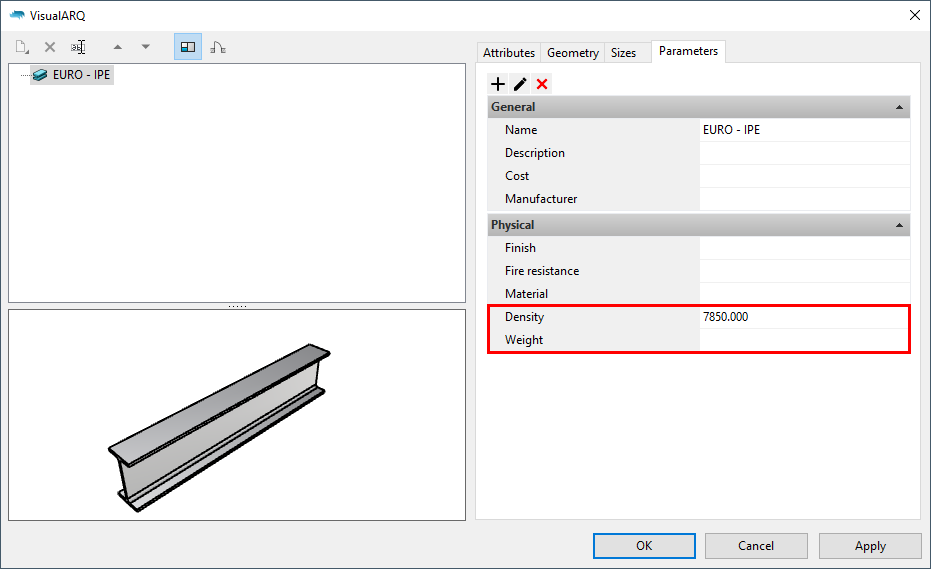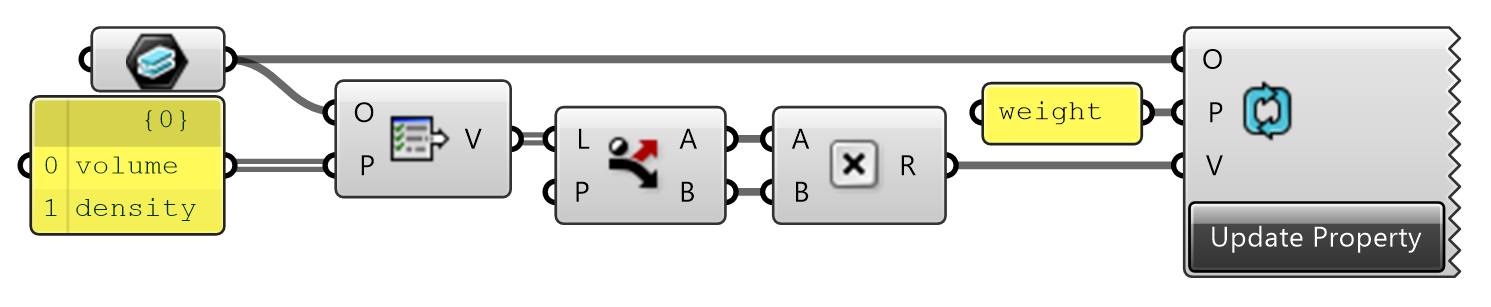Select Page

# How can I create calculated parameters with Grasshopper?

It is possible to create calculated parameters using the VisualARQ components in Grasshopper. Let’s learn how with this short example about how to calculate the weight of a beam from its volume and density.
Step 1. First of all, we need to have available all the parameters we are going to use. As the parameter volume is already created by default, we need to create the weight and density parameters. In this case, we will create them By Style. Lastly, we will assign a value to the density parameter, which is a constant.Step 2. Then, we can build the Grasshopper definition where we will need the Get Property component to retrieve the value of the volume and density parameters, multiply them and assign the result to the weight parameter using the Update Property component.